## PLL - Permuting Last Layer

There are 21 PLL Algorithms that are divided into the following categories:

• EDGES ONLY
• CORNERS ONLY
• SWAP ONE SET OF ADJACENT CORNERS
• SWAP ONE SET OF CORNERS DIAGONALLY
• DOUBLE SPINS

 EDGES ONLYUa (R U' R U) R U (R U' R' U') R2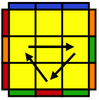Ub R2 U (R U R' U') (R' U') (R' U R')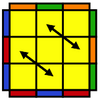Z (M2 U' M2 U')  (M' U2)  M2 U2 (M' U2)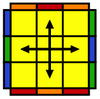H (M2  U' M2) U2 (M2  U' M2)
CORNERS ONLYAa x (R' U R') D2 (R U' R') (D2 R2)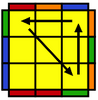Ab x (R2 D2) (R U R') D2 (R U' R)E x' (R U' R') D (R U R') D'

(R U R') D (R U' R') D'
SWAP ONE SET OF ADJACENT CORNERS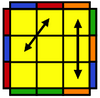Ra (R U' R' U') (R U R) D

(R' U' R) D' (R' U2 R' ) U'Rb (R' U2 )(R U2 ') (R' F)

(R U R' U')(R' F' R2 U')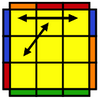Ja (R' U L' U2) (R U' R' U2 R) L  (U')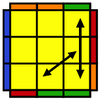Jb (R U R' F') (R U R' U')

R' F R2 U' R' ( U')T

(R U R' U') (R' F R2 U')

R' U' (R U R' F')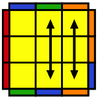F (R' U' F')(R U R' U')(R' F R2 U')

(R' U' R U)(R' U R)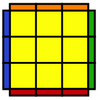SOLVED
SWAP ONE SET OF CORNERS DIAGONALLYV (R' U R' U') y (R' F' R2 U')

(R' U R' F)  (R F)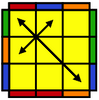Y F (R U' R' U') (R U R' F')

(R U R' U') (R' F R F')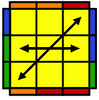Na (R U R'U) (R U R'F') (R U R'U')

(R'F R2 U') R' U2 (R U'R')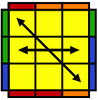Nb (R' U R U') (R' F' U' F) (R U R' F)

R' F' (R U' R)
DOUBLE SPINSGa R2 u (R' U R' U') (R u') R2 y' (R' U R)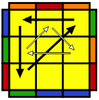Gb (F' U' F) (R2 u R' U) (R U' R u') R2'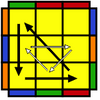Gc

R2 U' (R U' R U) (R' U R2 D')

(U R U' R') D U'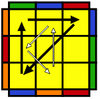Gd (R U R') y' (R2 u' R U') (R' U R' u) R2

Lookup all PLL Algorithms easily with CubeAlgs.com